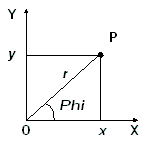# Point Object

Properties

Methods

## Summary

Point or vector in 2D space.

## Details

The Point object represents the point or vector in 2D space. It is used in many methods and properties where a pair of coordinates is needed.

To create a new Point object you can use the PointXY or PointRA methods of the Application object. Each of these creates a new Point with given Cartesian or polar coordinates. Once created, you can use a Point for several needs. You can change the Point's coordinates at any time using either Cartesian or polar coordinates or a mix.

The following example creates a new point, adds the vertex to the geometric model, then changes the point's coordinates and uses it for creating another vertex.X property as Double (read and write) Cartesian coordinates Y property as Double (read and write) R property as Double (read and write) Polar coordinates Phi property as Double (read and write)

The following example creates p as a new Point, sets its Cartesian coordinates as (x=0, y=1) and uses the point for creating a new model vertex. Then we change the Phi polar coordinate to 45 degree (the R polar coordinate remains unchanged) and use the point to create another model vertex.

Dim QF As QuickField.Application
Dim prb as Quickfield.Problem
Dim mdl as Quickfield.Model
.......
Dim p As QuickField.Point
Set p = QF.PointXY(0, 1)
With mdl.Shapes